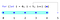# for loopsFor loops usually start counting from 0.

## Syntax

`for (initialize; condition; increment);for (initialize; condition; increment) single statement;for (initialize; condition; increment) { multiple; statements; }`

## 0-index based counter

`for (let i = 0;; i++) {    console.log("loop, i = " + i);    if (i > 1)        break;};`
`"loop, i = 0""loop, i = 1""loop, i = 2"`

## The Infinite for Loop

`for(;;) console.log("hi"); // Infinite for loop - don't do it`

## Multiple Statements

`let counter = 0;function inc() { counter++; }for (let i = 0; i < 10; i++, inc());console.log(counter); // 10`

## Increment Numbers

`let counter = 0;for (let i = 0; i < 10; i++)    counter++;counter; // 10;`

## Nested for Loops

`for (let y = 0; y < 2; y++)    for (let x = 0; x < 2; x++)        console.log(x, y);`
`0 01 00 11 1`

## Loop’s Length

`“loop.”“loop.”“loop.”`

`02`

## Breaking To A Label

`let c = 0;mark: for (let i = 0; i < 5; i++)    inner: for (let j = 0; j < 5; j++)        c++; if (i == 2) break mark;console.log(c); // 11`
`let c = 0;mark: for (let i = 0; i < 5; i++)    inner: for (let j = 0; j < 5; j++)        c++; if (i == 2) break inner;console.log(c); // 21`

## Breaking from a labeled non for-loop scope

`block: {    console.log("before");    break block;    console.log("after");}`
`"before"`

# for…of Loop

## for…of Loops And Generators

`// Generator function:function* generator() {  yield 1;  yield 2;  yield 3;}for (let value of generator())  console.log( value );`
`123`

## for…of Loops And Strings

`let string = 'text';for (let value of string)  console.log( value );`
`'t''e''x''t'`

## for…of Loops And Arrays

`let array = [0, 1, 2];`
`for (let value of array)   console.log( value );`
`012`

## for…of Loops And Objects (won’t work)

`let object = { a: 1, b: 2, c: 3 };for (let value of object) // Error: object is not iterable   console.log( value );`

## for…of loops and objects that were converted to iterables

`let enumerable = { property : 1, method : () => {} };for (let key of Object.keys( enumerable )) console.log(key);> property> methodfor (let value of Object.values( enumerable )) console.log(value);> 1> () => {}for (let entry of Object.entries( enumerable )) console.log(entry);> (2) ["prop", 1]> (2) ["meth", ƒ()]`

# for…in Loops

## for…in loops and enumerable object properties

`let object = { a: 1, b: 2, c: 3, method: () => { } };for (let value in object)    console.log(value, object[value]);`
`123() => { }`

# While Loops

`while (condition) { /* do something until condition is false */ }`
`let c = 0;while (c++ < 5)    console.log(c);`
`12345`
`while (condition_1) {    if (condition_2)        break;}`

## While and continue

`let c = 0;while (c++ < 1000) {    if (c > 1)        continue;    console.log(c);}`
`1`

# Arrays

## Array.forEach

`let fruit = ['pear', 'banana', 'orange', 'apple', 'pineapple'];let print = function(item, index, object) { console.log(item); }fruit.forEach( print );`
`fruit.forEach(function(item, index, object) {    console.log(item, index, object);});`
`fruit.forEach((item, index, object) => {    console.log(item, index, object);});`
`"pear",      0, (5)["pear","banana","orange","apple","pineapple"]"banana",    1, (5)["pear","banana","orange","apple","pineapple"]"orange",    2, (5)["pear","banana","orange","apple","pineapple"]"apple",     3, (5)["pear","banana","orange","apple","pineapple"]"pineapple", 4, (5)["pear","banana","orange","apple","pineapple"]`
`fruit.forEach(item => console.log(item));`
`"pear""banana""orange""apple""pineapple"`

## Array.every

`let numbers = [0,1,2,3,4,5,6,7];let result = numbers.every(value => value < 10 );result; // true`
`let numbers = [0,1,256,3,4,5,6,7];let result = numbers.every(value => value < 10 );result; // false`
`let numbers = [0,1,256,3,4,5,6,7];let result = numbers.every(value => value++ < 10 );console.log(result); // falseconsole.log(numbers); // Original array is unchanged`

## Array.some

`let numbers = [0,10,2,3,4,5,6,7];let condition = value => value < 10; // value is less than 10let some = numbers.some(condition); // truelet every = numbers.every(condition); // false`

## Array.filter

`let numbers = [0,10,2,3,4,5,6,7];let condition = value => value < 10;let filtered = numbers.filter(condition);console.log(filtered); // [0,2,3,4,5,6,7] // 10 is filtered out!console.log(numbers); // Original array remains unchanged`

## Array.map

`let numbers = [0,1,256,3,4,5,6,7];let result = numbers.map(value => value = value + 1 );console.log(result); // [1,2,257,4,5,6,7,8] // incremented by 1console.log(numbers); // Original array is unchanged`

## Array.reduce

`const R = (accumulator, currentValue) => accumulator + currentValue;`
`const numbers = [1, 2, 4];const R = (accumulator, currentValue) => accumulator + currentValue;console.log(numbers.reduce(R)); // 7`

## Creating Your Own Object Iterables With [Symbol.iterator]

`let iterable = {  [Symbol.iterator]() {    return {      i : 0,      next() {        if (this.i < 3)          return { value : this.i++, done : false }        return { value : 1, done : true }      }    }  }}for (let value of iterable)  console.log( value );`

# Not to be mistaken for Froot Loops

Issues. Every webdev has them. Published author of CSS Visual Dictionary https://amzn.to/2JMWQP3 few others…

## More from JavaScript Teacher

Issues. Every webdev has them. Published author of CSS Visual Dictionary https://amzn.to/2JMWQP3 few others…